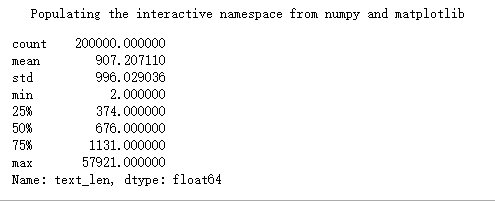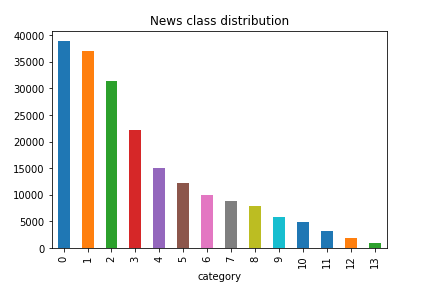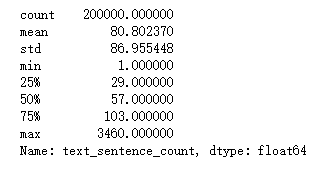## 数据读取

import pandas as pd


labeltext
22967 6758 339 2021 1854 3731 4109 3792 4149 15…
114464 486 6352 5619 2465 4802 1452 3137 5778 54…
37346 4068 5074 3747 5681 6093 1777 2226 7354 6…
27159 948 4866 2109 5520 2490 211 3956 5520 549…
33646 3055 3055 2490 4659 6065 3370 5814 2465 5…

## 数据分析

### 句子长度分布

%pylab inline
train_df['text_len']=train_df['text'].apply(lambda x:len(x.split(' ')))
train_df['text_len'].describe()### 新闻类别分布

train_df['label'].value_counts().plot(kind='bar')
plt.title('新闻类别分布')
plt.xlabel('category'){‘科技’: 0, ‘股票’: 1, ‘体育’: 2, ‘娱乐’: 3, ‘时政’: 4, ‘社会’: 5, ‘教育’: 6, ‘财经’: 7, ‘家居’: 8, ‘游戏’: 9, ‘房产’: 10, ‘时尚’: 11, ‘彩票’: 12, ‘星座’: 13}

### 字符分布

from collections import Counter
all_lines = ' '.join(list(train_df['text']))
word_count = Counter(all_lines.split(" "))
word_count = sorted(word_count.items(), key=lambda d:d, reverse = True)
print(len(word_count))
print(word_count[0:2])


import re
train_df['text_sentence']=train_df['text'].apply(lambda x:re.split('3750|900|648',x))
train_df['text_sentence_count']=train_df['text_sentence'].apply(lambda x:len(x))
print(train_df['text_sentence_count'].describe())## 总结

07-3061
07-2312207-22212
07-2298
07-2927
07-2331
08-0273
07-3187
07-2142
07-2257